# Linear Cryptanalysis Tutorial

This is going to be a fun tutorial; we're going to learn about a technique called linear cryptanalysis. There have been tons of papers about this category of cryptanalytic attacks since Matsui discovered it. However, I challenge anyone to find one that doesn't require a PhD to understand. I have slowly been learning the language of the mathematicians and academic crypto-folks and I hope to present this idea in a way that it is accessible and understandable. I'm going to assume a basic familiarity with how typical ciphers are put together and what some terms mean. Reading through my page on Block Ciphers should get you up to speed. If you're having trouble understanding something here, please write me an email and I'll do my best. I'm still a beginner at this stuff, but I feel I've got a firm grasp of how this attack works.

Source Code - This program, written in C, implements the toy cipher, calculates the approximations, uses linear cryptanalysis to break the key, and finally compares the computational work to brute force.

### What's the Problem?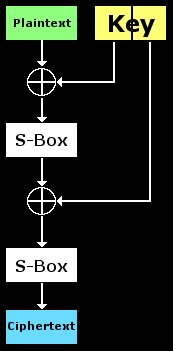Alrighty, first we're going to discuss why one needs an attack like this. To the right is a diagram of our toy cipher that we'll eventually break. As you can see, it consists of two rounds. In each round, the input is XOR'd with a chunk of the key and then ran through a Substitution Box. Keep in mind that we have 16 pairs of known-plaintext/ciphertext. By this, I mean that 16 inputs to this cipher are known to us along with their encrypted output. All of these known pairs were encrypted with the same key. We also know how the S-Box operates completely; the only thing unknown is the key. We're going to use this information, some statistical information about the S-Box, and some test encryptions to recover the key with far less work than brute forcing every possible key.

Imagine if only the left half of the key was used and there was only one round in this cipher. We could trivially break it as follows: Run the ciphertext backward through the S-Box and then XOR it with the plaintext. This gives us the key with no effort and only one known pair. Now what happens if we try a similar technique on the full two-round version? Lets walk through it: first we run the ciphertext through the 2nd S-Box backwards, then we XOR with the input to round #2...Wait a minute, we don't know what the input to round #2 is! In order to get that information, we must know the output of round #1 and thus the left-half of the key.

Ok, maybe we can recover the left half of the key and then go for the right half. So we take our known plaintext and XOR it with a left-key-half guess. Next we run it through the first S-Box and we have a guess at the output of round #1. But we don't have anything to compare this output to. We have no idea if its correct and thus can't use it for anything. Try to find a way to recover the key without testing every single key (brute force). Just trying different simple ideas and seeing why they won't work is really the only way to see that another solution is necessary. Play with that diagram until it makes complete sense that the full 2-round cipher is much harder to crack than the 1-round version.

### Toy Cipher Details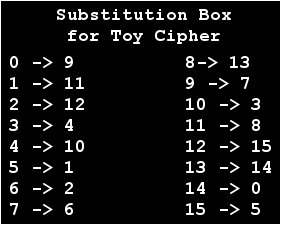Next, we'll define some of the parameters that the toy cipher uses. These will be kept low for a variety of reasons. By making this algorithm use small blocks and keys, any software written to test things out will be fast and give us instant results. Also processing data a few bits at a time makes things easier to understand and follow in my opinion. This cipher's parameters can be grown later for a more real-world test if desired. The block size is only 4 bits and the key size is only 8 bits (2x 4 bit subkeys). This means that each 4-bit block is mixed with the 4-bit left-half of the key in round 1 and then mixed with the 4-bit right-half in round 2. There are only 256 possible keys and 16 possible inputs/outputs. As you'll find this means that multiple keys can encrypt a plaintext block into the same ciphertext block. The trick is finding the correct key that will do this for all known pairs.

Also take note that the diagram to the left has been altered slightly. We will no longer refer to the parts of the key as "left-half" and "right-half". The left part of the key has been renamed K1 and the right K2. These pieces of the original key are known as subkeys and are the target. By recovering K1 and K2, we will also recover the original key. Also, if it is no clear, each subkey is 4 bits long. Also, the data in-between rounds 1 and 2 has been labelled "M" which stands for midpoint. We do not know this value yet, but it is a very important place in the cipher. Think of M as being both the output of round #1 and the input to round #2.

Now a quick word on the S-Boxes. They are both identical; this is not required but it makes things simpler to code and think about. The listing to the right shows the contents of the S-Box. It simply takes an input shown to the left side of the arrow and outputs the cooresponding value on the right side of the arrow.

### Linear Approximations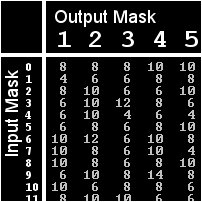You know that we can make a random guess at K0 and thus a random guess at M. What if there was a way to test if M is correct? We only have known plaintext(P) and known ciphertext(C); we don't have known-midpoint(M). Well, there is no way to know for sure if our guessed-M is correct (without brute forcing of course), but there is a way to make an educated guess. We don't even have to have any information about K1 to do it! Before we delve into this topic, its important to understand why being able to validate M is helpful to the attacker. If we know with 90% certainty that M is correct after guessing K0, we can run the ciphertext backwards through S-Box #2 and then XOR it with the guessed-M. This would give us a good guess at K1. If we can accomplish this then we would be left with a guess for K0 and a guess for K1 that are both correct with a probability of 90%. This educated guess at the key can then be plugged into the cipher and tested against all of the known pairs. If they all encrypt correctly using K0 and K1, then they are correct and you win.

Linearity is a difficult and ambiguous topic to grasp and I do not possess the mathematical prowess to do it justice. Instead, I will explain my layman's idea of what it is. If you feed a random input with a particular property into a magic box and can guess the cooresponding property in the output, the magic box is at least somewhat linear. For example, imagine that your box takes an input and adds 1 to it. Now lets say that the property you are looking for is whether the input/output is even. By feeding it an input, you know that this property will be the opposite in the output every single time. In other words, adding 1 to an even number with always produce an odd number and vise-versa. This magic box would be completely linear in regard to divisibility by two. This way of thinking about it may not be completely correct but it helps to understand the concept especially as it applies to linear cryptanalysis.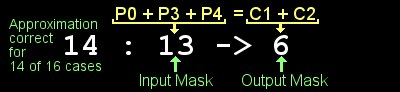S-Boxes are used to add non-linearity to ciphers. Ideally, an S-Box should receive an input with property X and output a number that has property Y exactly 50% of the time. This does not mean there is anything tricky going on in there, it just means that exactly half of those property X inputs should output a property Y output. Lets go ahead and talk about the property we'll be using: parity. Parity is a boolean value (1 or 0) that we get if we XOR together some of the bits of a number. The bits that we XOR together are defined by another number called a mask. If you want to figure out the parity of a number when masked; do a bitwise AND operation on the mask and the value. Then XOR all of the bits in this result together. The mask tells us to ignore certains bits of the number when calculating the parity.

### Best Approximation?

Ok, so now you have this table of numbers and their associated mask pairs. What do you do with it? We haven't touched any plaintext/ciphertext pairs yet; only crunched numbers related to the S-Box. Lets pretend that one of those approximations in the table read 16; that would mean that it held 100% of the time. In other words, no matter what input you feed that S-Box, its masked input parity will equal the cooresponding masked output parity after being run through the S-Box. Something to note here is that an XOR does not impact this fact at all. If every input is XOR'd by some value (like a subkey), the most impact it will have it flipping these odds. If you XOR the input by 1 before masking, it may make the approximation hold true 0% of the time instead of 100%.

One of the good linear approximations in the table for the toy cipher's S-Box is 11->11 which holds true for 14/16 inputs. In other words, if you XOR bits 1, 2, and 4 of almost any input, this value will equal the cooresponding output's parity (using the same mask in this case). This fact will hold true for all but 2 inputs. If these inputs are first XOR'd by a subkey, this probability of truth may become 2/16.

### Testing the Midpoint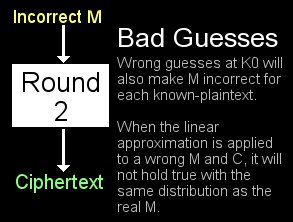Remember when I said we can test if M is correct after guessing K0? Do not think of M as the output of round 1 but rather the input of round 2. Remember that round 2 consists of an XOR with K1 that doesnt really affect the probability of a linear approximation holding true. And of course, then comes the S-Box which we have now approximated. So we'll take our guessed inputs and use the input mask of 11 to get their parity. Next take the cooresponding REAL outputs of round 2 (known ciphertexts) and get their parity when masked with 11. If these guessed input parities are equal to the real output parities for around 14/16 or 2/16 of the known pairs, the K0 that was used to generate those M values is likely correct.

Notice that we don't really care how M was generated except that we guessed K0 to get it. The contents of round 1's S-Box don't matter and are not being tested by the linear approximation. Its only the characteristics of round #2's S-Box that are being used to test if the Ms are likely correct. If the guessed K0 and thus Ms are not correct, then the linear approximation will not be true with the same probability as the real deal. In other words, we are taking our known outputs and testing them against guessed inputs. The linear approximation is what allows us make an educated guess that the input generated this output. Because we guessed at K0 to get this input, if the approximation holds true with the right probability it means that the guessed K0 is likely correct. Now that we know P, K0, M, and C; some basic fiddling will get us K1. Just take C and feed it through the S-Box backwards and XOR it with the now-known M. This will give us K1 and, when combined with K0, leaves us with the full key. Finally, we test this full guessed key against every known-plaintext and see if it generates the cooresponding ciphertext. If it does, then we have found the key!

### Implementation and Summary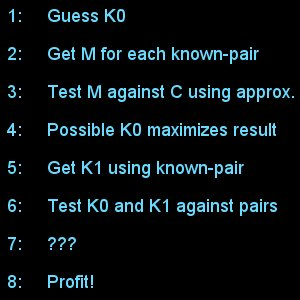If we want to test this out with real code (something the internet is seriously lacking regarding this stuff); we need to take it in steps. Step 1: Find a good linear approximation for the S-Box. We mask every possible 4-bit input with every possible input and compare the parity against the parity of the output masked by every possible output mask. This concept is much to understand when you check out the source code. This step makes us perform 16 * 16 * 16 tests (inputs, input masks, output masks). For how small the keyspace is, this is a lot of work. Remember though, we only need to do this once as long as the S-Box doesn't change. Now we have the percentage of inputs that a input/output mask pair holds true for. We start looking for pairs that hold true close to 100%. You'll find some good candidates that hold true for 14 of the 16 possible inputs. I use the input mask of 11 and the output mask of 11.

Now that we have an approximation to test for, guess every value of K0 and encrypt(through round 1) all 16 known plaintexts with it to get 16 guesses at M. Then test each of these Ms against the linear approximation (used in round 2). The series of Ms that either old true almost none of the time or almost all of the time makes the K0 that generated them possibly correct. By doing a little math on the results (subtract 8 and square the result), you can give each candidate K0 a "score". Then find the highest score and make a list of the K0 candidates that have it. In this implementation, I find that there are usually 2 but sometimes 0 or even 4.

So now you have a list of candidate K0s; use one of the known pairs to calculate a cooresponding candidate K1. Next test this educated key guess against all known plaintext/ciphertext pairs. If it matches then you've found the key. My program also keep track of how many total calculations this whole process takes as well as how many it took before a key was found. It then brute forces the whole thing and does the same type of counting. This lets you compare how much work was saved by not doing brute force.

### Results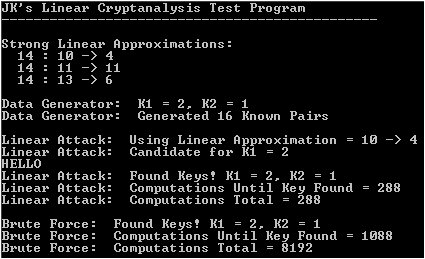So how well does method work and how does it compare to brute force? I use the metric of how many times the round function is called. So, by doing this, testing every key against 16 known plaintext/ciphertext pairs would take 256 * 16 * 2 computations (2 rounds). Usually the linear attack finds 2 candidate K0s; the more it finds, the more it must test to prove/disprove (against all known-pairs). The linear attack must test every K0 against all known-pairs to get those guesses at M. So to get the scores for K0, it must do 16 * 16 computations. Then it tests each likely candidate (assume 2) against all known-pairs (using K0 guess to calculate K1). This adds 2 * 2 * 16 computations (2 rounds, 2 candidates, 16 pairs). So as far as total computations (times the round function is called), brute forcing ends up around (256 * 16 * 2) and this linear attacks lands at around (16 * 16 + 16 * 2 * 2).

That was total computations for each method and the linear attack is well-ahead. Another standard for measuring sucess is how many computations it takes to actually find the key. Typically, brute force will find the key after testing around 4000 keys (midpoint of the total). The linear method usually finds the key after around 300 computations. The maximum depends on the number of candidate K0s found; for 2 candidates, the max is 320. So compare running the round function an average of 4000 times to running is 300 times and its obvious that the linear attack works. Now, it doesn't always work though, sometimes it doesnt find the correct key at all. Some basic experimental testing shows that it does find it around 80% of the time, but this is just observing runs and counting successes. Also, in order to pull this off, you must precalculate that 16 * 16 table of input/output masks for the target S-Box.